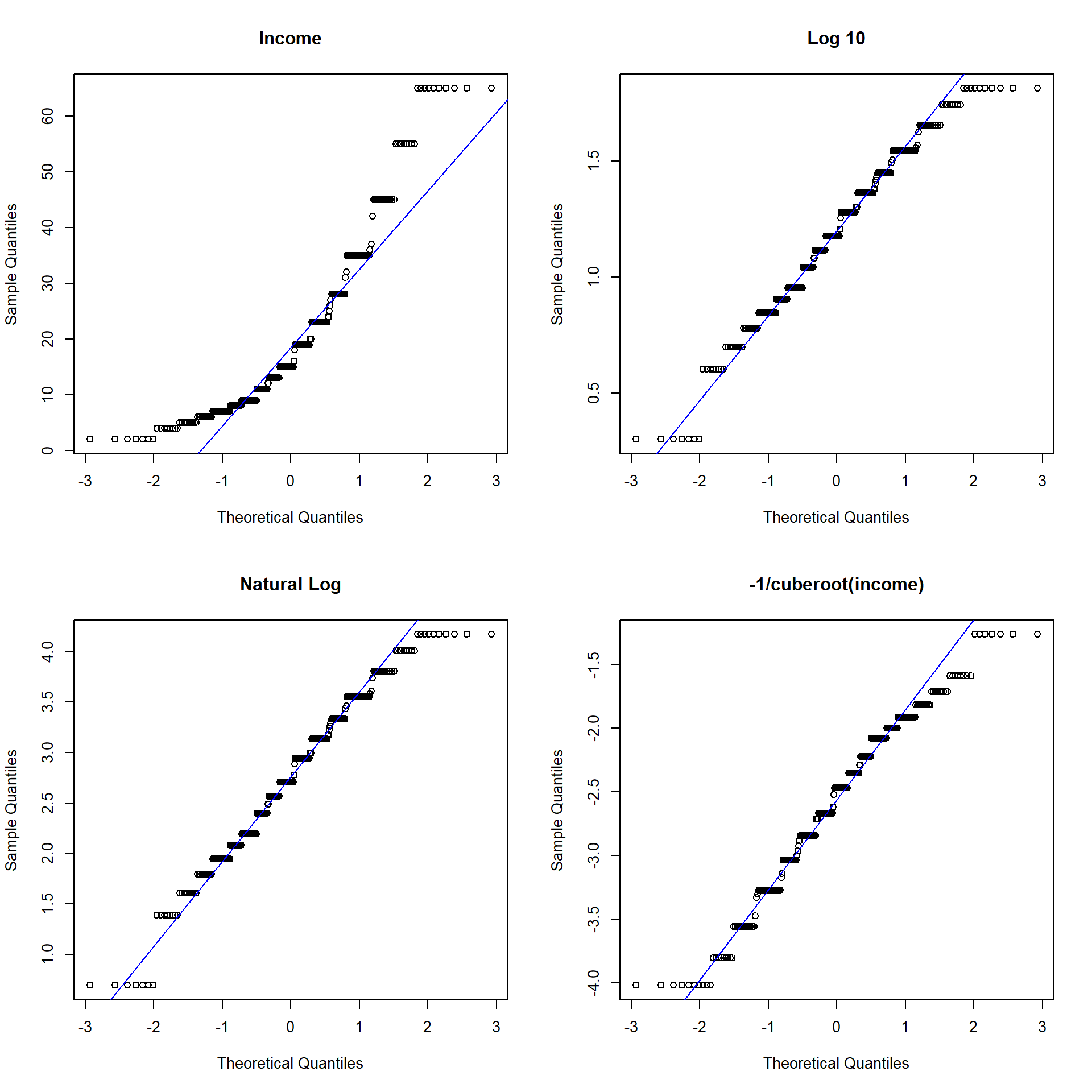## 1.3 Data Management

Questions to ask yourself (and the data) while reviewing the codebook to choose variables to be used in an analysis.

1. Do you need to code out missing data?
• Missing values sometimes are recorded as something like MISSING or -99?
2. Do you need to make response codes more logical?
• Some systems will record 1=YES and 2=NO. This should be changed to 0=NO.
3. Do you need to recode numerical variables to categorical?
• Sometimes categorical data will be recorded as 1, 2, 3 etc when those numbers represent named categories.
4. Do you need to create secondary variables?
• Such as an average across measures to create a score.

Some of these answers will come only after you look at your data. This can be looking at the raw data itself but also looking at tables and charts generated from the data. Often when you try to create a plot or table you will encounter an error or something odd looking that will be the notification that something has to be adjusted.

Let’s look at a few of the common data management processes.

### 1.3.1 Missing data

In Excel, missing data can show up as a blank cell. In SPSS it is represented as a . period. R displays missing data as NA values.

Missing Data in SPSS: https://stats.idre.ucla.edu/spss/modules/missing-data/

Why would data be missing? Other than the obvious data entry errors, tech glitches or just non-cooperative plants or people, sometimes values are out of range and you would rather delete them than change their value (data edit).

Lets look at the religion variable in the depression data set.

table(depress$relig, useNA="always") ## ## 1 2 3 4 6 <NA> ## 155 51 30 56 2 0 Looking at the codebook, there is no category 6 for religion. Let’s change all values to NA. depress$relig[depress$relig==6] <- NA This code says take all rows where relig is equal to 6, and change them to NA. Confirm recode. table(depress$relig, useNA="always")
##
##    1    2    3    4 <NA>
##  155   51   30   56    2

Notice the use of the useNA="always" argument. If we just looked at the base table without this argument, we would have never known there was missing data!

table(depress$relig) ## ## 1 2 3 4 ## 155 51 30 56 What about continuous variables? Well there happens to be no other missing data in this data set, so let’s make up a set of 7 data points stored in a variable named y. y <- c(1, 2, 3, NA, 4, NA, 6) y ##  1 2 3 NA 4 NA 6 The #1 way to identify missing data in a continuous variable is by looking at the summary() values. mean(y) ##  NA summary(y) ## Min. 1st Qu. Median Mean 3rd Qu. Max. NA's ## 1.0 2.0 3.0 3.2 4.0 6.0 2 mean(y, na.rm=TRUE) ##  3.2 In R, any arithmetic function (like addition, multiplication) on missing data results in a missing value. The na.rm=TRUE toggle tells R to calculate the complete case mean. This is a biased measure of the mean, but missing data is a topic worthy of it’s own course. ### 1.3.2 Identifying Variable Types The str function is short for structure. This shows you the variable names, what data types R thinks each variable are, and some of the raw data. You can also use the view() function to open the data as a similar spreadsheet format, or head() to see the top 6 rows of the data. The latter is sometimes less than helpful for a very large data set. str(depress) ## 'data.frame': 294 obs. of 37 variables: ##$ id      : int  1 2 3 4 5 6 7 8 9 10 ...
##  $sex : int 2 1 2 2 2 1 2 1 2 1 ... ##$ age     : int  68 58 45 50 33 24 58 22 47 30 ...
##  $marital : int 5 3 2 3 4 2 2 1 2 2 ... ##$ educat  : int  2 4 3 3 3 3 2 3 3 2 ...
##  $employ : int 4 1 1 3 1 1 5 1 4 1 ... ##$ income  : int  4 15 28 9 35 11 11 9 23 35 ...
##  $relig : int 1 1 1 1 1 1 1 1 2 4 ... ##$ c1      : int  0 0 0 0 0 0 2 0 0 0 ...
##  $c2 : int 0 0 0 0 0 0 1 1 1 0 ... ##$ c3      : int  0 1 0 0 0 0 1 2 1 0 ...
##  $c4 : int 0 0 0 0 0 0 2 0 0 0 ... ##$ c5      : int  0 0 1 1 0 0 1 2 0 0 ...
##  $c6 : int 0 0 0 1 0 0 0 1 3 0 ... ##$ c7      : int  0 0 0 0 0 0 0 0 0 0 ...
##  $c8 : int 0 0 0 3 3 0 2 0 0 0 ... ##$ c9      : int  0 0 0 0 3 1 2 0 0 0 ...
##  $c10 : int 0 0 0 0 0 0 0 0 0 0 ... ##$ c11     : int  0 0 0 0 0 0 0 0 0 0 ...
##  $c12 : int 0 1 0 0 0 1 0 0 3 0 ... ##$ c13     : int  0 0 0 0 0 2 0 0 0 0 ...
##  $c14 : int 0 0 1 0 0 0 0 0 3 0 ... ##$ c15     : int  0 1 1 0 0 0 3 0 2 0 ...
##  $c16 : int 0 0 1 0 0 2 0 1 3 0 ... ##$ c17     : int  0 1 0 0 0 1 0 1 0 0 ...
##  $c18 : int 0 0 0 0 0 0 0 1 0 0 ... ##$ c19     : int  0 0 0 0 0 0 0 1 0 0 ...
##  $c20 : int 0 0 0 0 0 0 1 0 0 0 ... ##$ cesd    : int  0 4 4 5 6 7 15 10 16 0 ...
##  $cases : int 0 0 0 0 0 0 0 0 1 0 ... ##$ drink   : int  2 1 1 2 1 1 2 2 1 1 ...
##  $health : int 2 1 2 1 1 1 3 1 4 1 ... ##$ regdoc  : int  1 1 1 1 1 1 1 2 1 1 ...
##  $treat : int 1 1 1 2 1 1 1 2 1 2 ... ##$ beddays : int  0 0 0 0 1 0 0 0 1 0 ...
##  $acuteill: int 0 0 0 0 1 1 1 1 0 0 ... ##$ chronill: int  1 1 0 1 0 1 1 0 1 0 ...

Right away this tells me that R thinks all variables are numeric integers, not categorical variables. This will have to be changed. We’ll get to that in a moment.

In SPSS you’ll the following set of icons to tell you what data types the program thinks each column is:Consider the variable that measures marital status.

table(depress$marital) ## ## 1 2 3 4 5 ## 73 127 43 13 38 str(depress$marital)
##  int [1:294] 5 3 2 3 4 2 2 1 2 2 ...
class(depress$marital) ##  "integer"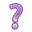What data type does R see this variable as? When variables have numerical levels it is necessary to ensure that the program knows it is a factor variable. The following code uses the factor() function to take the marital status variable and convert it into a factor variable with specified labels that match the codebook. depress$marital <- factor(depress$marital, labels = c("Never Married", "Married", "Divorced", "Separated", "Widowed")) It is important to confirm the recode worked. If it did not you will have to re-read in the raw data set again since the variable marital was replaced. table(depress$marital)
##
## Never Married       Married      Divorced     Separated       Widowed
##            73           127            43            13            38
class(depress$marital) ##  "factor" ### 1.3.3 Outliers Let’s look at the age variable in the depression data set. par(mfrow=c(1,2)) boxplot(depress$age)
hist(depress$age)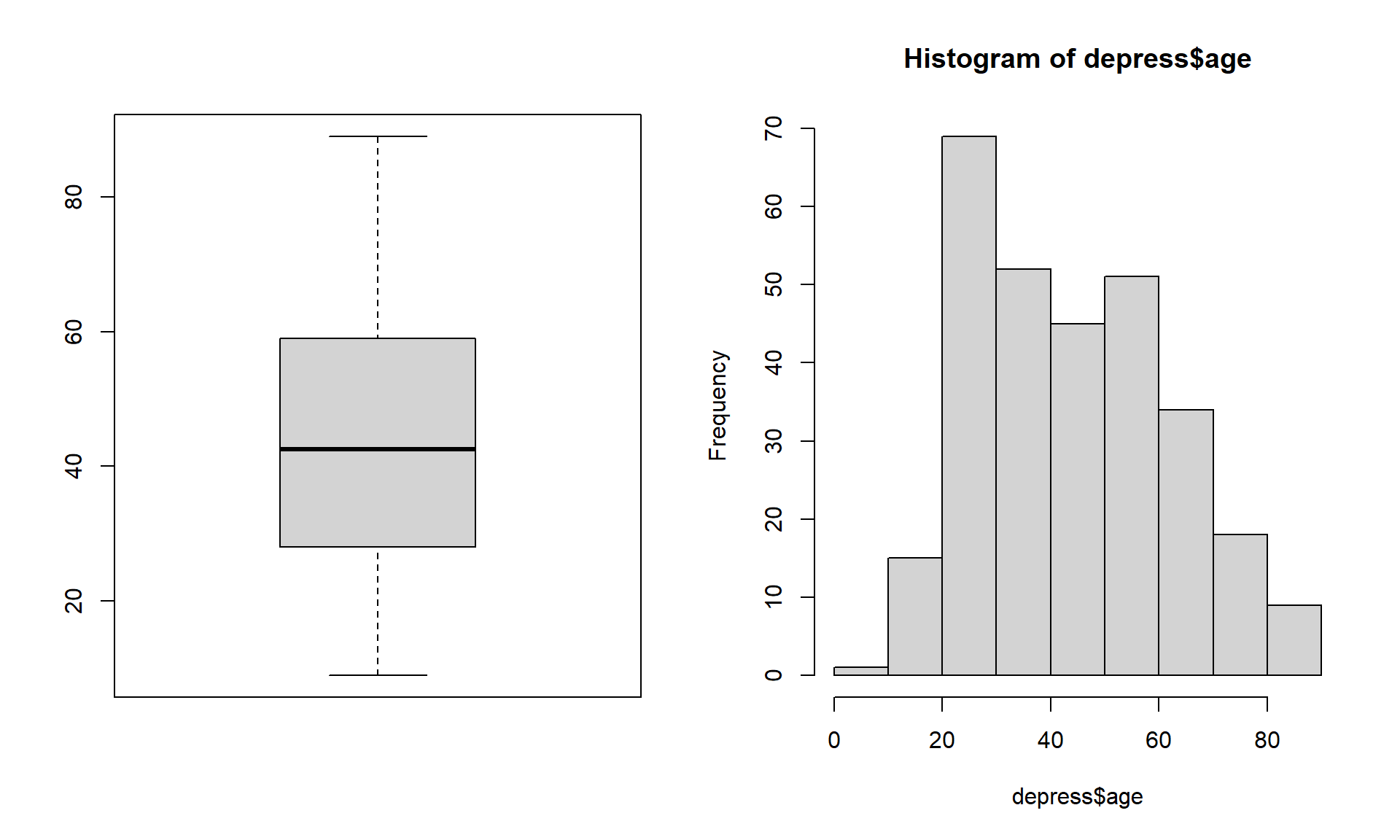Just looking at the data graphically raises no red flags. The boxplot shows no outlying values and the histogram does not look wildly skewed. This is where knowledge about the data set is essential. The codebook does not provide a valid range for the data, but the description of the data starting on page 3 in the textbook clarifies that this data set is on adults. In the research world, this specifies 18 years or older. Now look back at the graphics. See anything odd? It appears as if the data go pretty far below 20, possibly below 18. Let’s check the numerical summary to get more details. summary(depress$age)
##    Min. 1st Qu.  Median    Mean 3rd Qu.    Max.
##    9.00   28.00   42.50   44.38   59.00   89.00

The minimum value is a 9, which is outside the range of valid values for this variable. This is where you, as a statistician, data analyst or researcher goes back to the PI and asks for advice. Should this data be set to missing, or edited in a way that changes this data point into a valid piece of data.

Another visual way to look for outliers in a continuous measurement is to create a boxplot. Here we look at boxplots of income across marital status category.

ggplot(depress, aes(y=income, x=marital)) + geom_boxplot()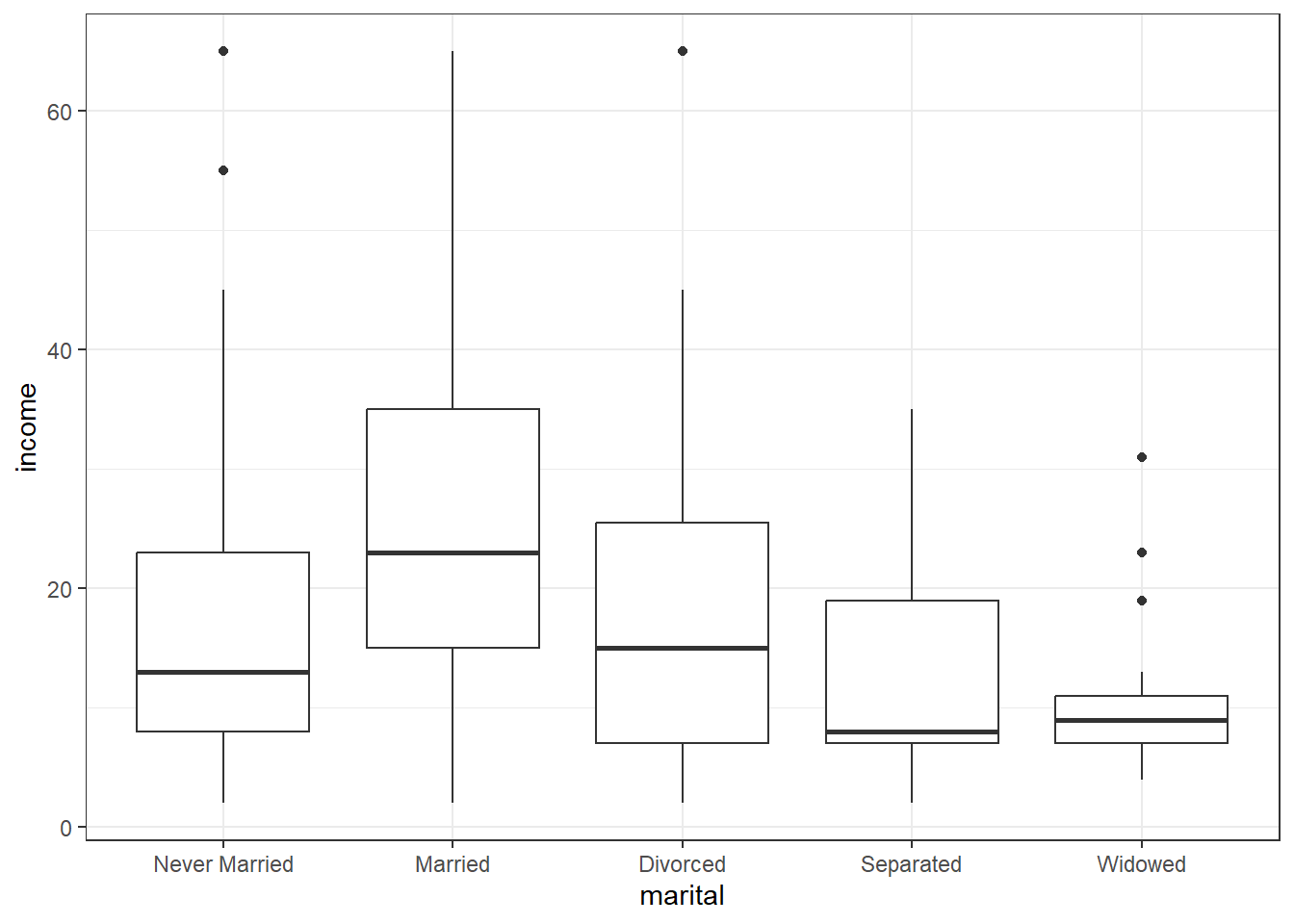While there are a few potential outliers (denoted by the dots), there are none so far away from the rest of the group (or at values such as 99 or -99 that may indicate missing codes) that we need to be concerned about.

As an example of a common data entry error, and for demonstration purposes, I went in and changed a 19 to a 9. So the correct thing to do here is to change that 9, back to a 19. This is a very good use of the ifelse() function.

depress$age <- ifelse(depress$age==9, 19, depress$age) The logical statement is depress$age9. Wherever this is true, replace the value of depress$age with 19, wherever this is false then keep the value of depress$age unchanged (by “replacing” the new value with the same old value).

Alternatively, you can change that one value using bracket notation. Here you are specifying that you only want the rows where age==9, and directly assign a value of 19 to those rows.

depress$age[depress$age==9] <- 19

Confirm the recode.

table(depress$marital, marital2, useNA="always") ## marital2 ## Married Never Married Sep/Div Widowed <NA> ## Never Married 0 73 0 0 0 ## Married 127 0 0 0 0 ## Divorced 0 0 43 0 0 ## Separated 0 0 13 0 0 ## Widowed 0 0 0 38 0 ## <NA> 0 0 0 0 0 This confirms that records where marital (rows) is Divorced or Separated have the value of Sep/Div for marital2 (columns). And that no missing data crept up in the process. Now I can drop the temporary marital2 variable and actually fix marital. (keeping it clean) depress$marital <- recode(depress$marital, "'Divorced' = 'Sep/Div'; 'Separated' = 'Sep/Div'") rm(marital2) #### 1.3.4.2 Binning a continuous variable into categorical ranges. Let’s create a new variable that categorizes income into the following ranges: <30, [30, 40), [40,50), [50, 60), 60+. The easiest way is to use the cut2 function in the package Hmisc. Note you don’t have to load the package fully to use a function from within that package. Useful for times when you only need to use a function once or twice. depress$inc_cut <- Hmisc::cut2(depress$income, cuts=c(30,40,50,60)) table(depress$inc_cut)
##
## [ 2,30) [30,40) [40,50) [50,60) [60,65]
##     231      28      16       9      10

#### 1.3.4.3 Dichotomizing a measure into 2 categories

Dichotomous variables tend to be binary indicator variables where a code of 1 is the level you’re interested in.

For example, gender is coded as 2=Female and 1=Male. This is in the right direction but it needs to be 0/1.

depress$sex <- depress$sex -1
table(depress$sex) ## ## 0 1 ## 111 183 0/1 binary coding is mandatory for many analyses. One simple reason is that now you can calculate the mean and interpret it as a proportion. mean(depress$sex)
##  0.622449

62% of individuals in this data set are female.

Sometimes the data is recorded as 1/2 (Yes/No), so just subtracting from 1 doesn’t create a positive indicator of the variable. For example, drink=1 if they are a regular drinker, and drink=2 if they are not. We want not drinking to be coded as 0, not 2.

table(depress$drink) ## ## 1 2 ## 234 60 The ifelse() function says that if depress$DRINK has a value equal to 2 ==2, then change the value to 0. Otherwise leave it alone.

depress$drink <- ifelse(depress$drink==2, 0, depress$drink) table(depress$drink)
##
##   0   1
##  60 234

#### 1.3.4.4 Sum or Average values across multiple variables

The Center for Epidemiological Studies Depression Scale (CESD) is series of questions asked to a person to measure their level of depression. CESD is calculated as the sum of all 20 component variables, and is already on this data set. Let’s create a new variable named sleep as subscale for sleep quality by adding up question numbers 5, 11, and 19.

Reference: http://cesd-r.com/cesdr/

depress$sleep <- depress$c5 + depress$c11 + depress$c19
## # depress <- depress %>% mutate(sleep = c5+c11+c19) # Not run. dplyr example
summary(depress$sleep) ## Min. 1st Qu. Median Mean 3rd Qu. Max. ## 0.000 0.000 1.000 1.167 2.000 7.000 #### 1.3.4.5 Transformations for Normality Let’s look at assessing normal distributions using the cleaned depression data set. hist(depress$income, prob=TRUE, xlab="Annual income (in thousands)",
main="Histogram and Density curve of Income", ylab="")
lines(density(depress$income), col="blue")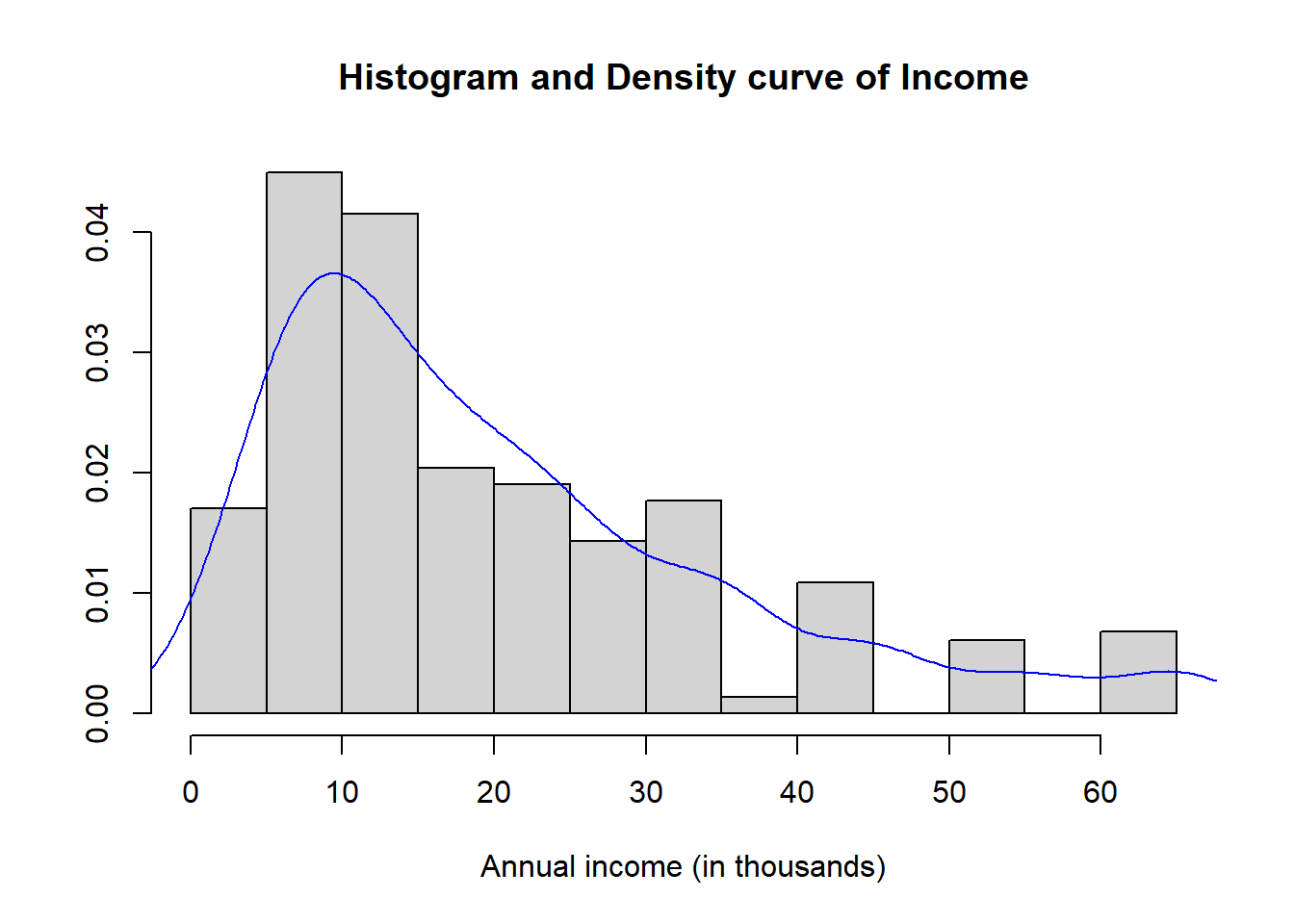summary(depress$income)
##    Min. 1st Qu.  Median    Mean 3rd Qu.    Max.
##    2.00    9.00   15.00   20.57   28.00   65.00

The distribution of annual income is slightly skewed right with a mean of $20.5k per year and a median of$15k per year income. The range of values goes from $2k to$65k. Reported income above $40k appear to have been rounded to the nearest$10k, because there are noticeable peaks at $40k,$50k, and $60k. In general, transformations are more effective when the the standard deviation is large relative to the mean. One rule of thumb is if the sd/mean ratio is less than 1/4, a transformation may not be necessary. sd(depress$income) / mean(depress$income) ##  0.743147 Alternatively Hoaglin, Mosteller and Tukey (1985) showed that if the largest observation divided by the smallest observation is over 2, then the data may not be sufficiently variable for the transformation to be decisive. max(depress$income) / (min(depress$income)+.1) ##  30.95238 Note these rules are not meaningful for data without a natural zero. Another common method of assessing normality is to create a normal probability (or normal quantile) plot. qqnorm(depress$income);qqline(depress$income, col="red")The points on the normal probability plot do not follow the red reference line very well. The dots show a more curved, or U shaped form rather than following a linear line. This is another indication that the data is skewed and a transformation for normality should be created. • Create three new variables: log10inc as the log base 10 of Income, loginc as the natural log of Income, and xincome which is equal to the negative of one divided by the cubic root of income. log10inc <- log10(depress$income)
loginc   <- log(depress$income) xincome <- -1/(depress$income)^(-1/3)
• Create a single plot that display normal probability plots for the original, and each of the three transformations of income. Use the base graphics grid organizer par(mfrow=c(r,c)) where r is the number of rows and c is the number of columns. Which transformation does a better job of normalizing the distribution of Income?
par(mfrow=c(2,2)) # Try (4,1) and (1,4) to see how this works.
qqnorm(depress$income, main="Income"); qqline(depress$income,col="blue")
qqnorm(log10inc, main="Log 10"); qqline(log10inc, col="blue")
qqnorm(xincome, main="-1/cuberoot(income)"); qqline(xincome, col="blue")